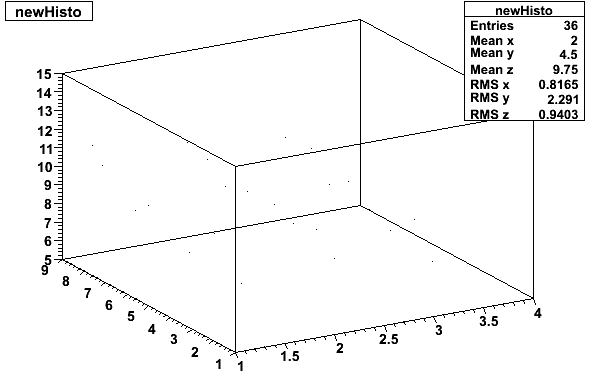# TH3D histo

Hi

I am having trouble plotting a TH3D histogram. I can write in the data but the histogram seems to show just a few small dots, it does seem to be plotting something but im not sure what.

I what to plot three arrays of data so x[n], y[n] against a third array value[n].
the code is simple, and I have attached the plot. Can someone see why it is not plotting correctly?

TH3D *newHisto = new TH3D(“newHisto”,“newHisto”,36,1,4,36,1,9,36,5,15);

int again=0;
int n;
for (int i=0; i<4;i++)
{
for (int j=0; j<9; j++)
{
n = (j+1) + (9*again);
x[n] = i+1;
y[n] = j+1;
value[n] = array[j][i];
cout<<"n= “<<n<<” x = “<<x[n]<<” y = “<<y[n]<<” value = "<<value[n]<<endl;
newHisto->Fill(x[n],y[n],value[n]);
}
again++;
}

TCanvas *c7 = new TCanvas(“c7”,“Loop temp Vs Phi”,100,20,600,400);
c7->SetBorderMode(0);
//gStyle->SetHistFillColor(kBlue);
gStyle->SetCanvasColor(0);
gStyle->SetOptFit(1111);
newHisto->UseCurrentStyle();

newHisto->Draw();
c7->SaveAs(“newHisto.eps”);

Thanks
knewHisto.ps (20.1 KB)

see class TGraph2D and also \$ROOTSYS/tutorials/graphs/graf2derrorsfit.C

Rene